# Python时间序列数据分析--以示例说明

https://www.analyticsvidhya.com/blog/2016/02/time-series-forecasting-codes-python/ 英文不错的读者可以前去阅读原文。

### 导读

1. 用pandas处理时序数据
2. 怎样检查时序数据的稳定性
3. 怎样让时序数据具有稳定性
4. 时序数据的预测

### 1. 用pandas导入和处理时序数据

import pandas as pd
import numpy as np
import matplotlib.pylab as plt
from matplotlib.pylab import rcParams
#rcParams设定好画布的大小
rcParams['figure.figsize'] = 15, 6


http://github.com/aarshayj/Analytics_Vidhya/tree/master/Articles/Time_Series_Analysis 中下载

data = pd.read_csv(path+"AirPassengers.csv")
print '\n Data types:'
print data.dtypes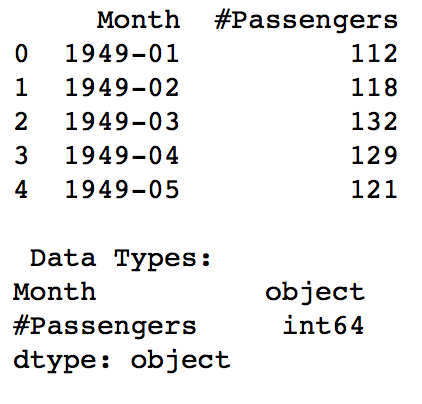dateparse = lambda dates: pd.datetime.strptime(dates, '%Y-%m')
#---其中parse_dates 表明选择数据中的哪个column作为date-time信息，
#---index_col 告诉pandas以哪个column作为 index
#--- date_parser 使用一个function(本文用lambda表达式代替)，使一个string转换为一个datetime变量
data = pd.read_csv('AirPassengers.csv', parse_dates=['Month'], index_col='Month',date_parser=dateparse)
print data.index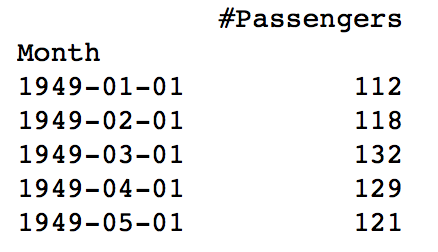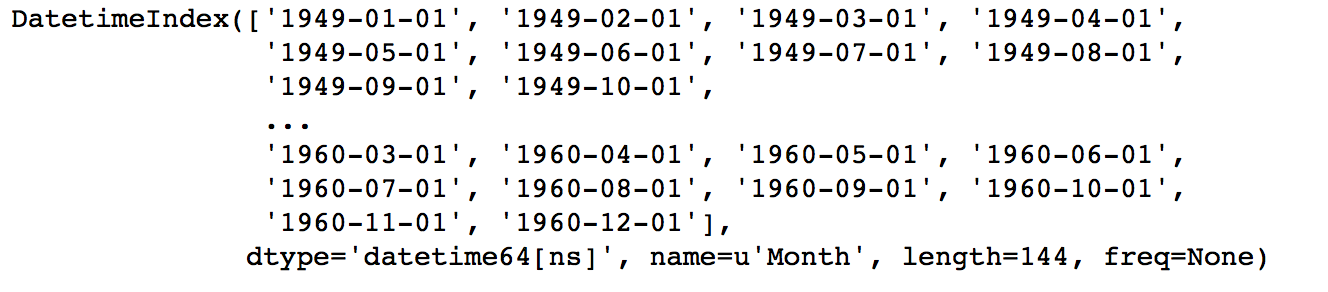### 2.怎样检查时序数据的稳定性(Stationarity)

#### 1. 判断数据是稳定的常基于对于时间是常量的几个统计量：

1. 常量的均值
2. 常量的方差
3. 与时间独立的自协方差

1. 均值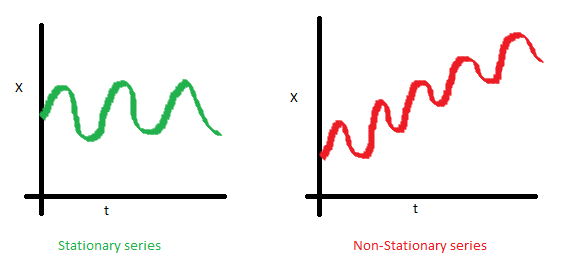X是时序数据的值，t是时间。可以看到左图，数据的均值对于时间轴来说是常量，即数据的均值不是时间的函数,所有它是稳定的；右图随着时间的推移，数据的值整体趋势是增加的，所有均值是时间的函数，数据具有趋势，所以是非稳定的。
2. 方差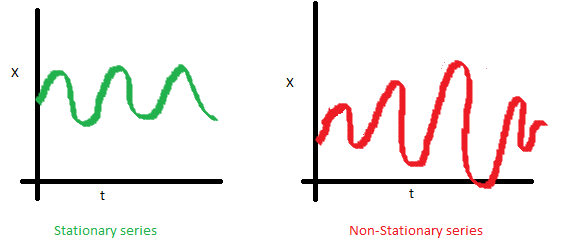可以看到左图，数据的方差对于时间是常量，即数据的值域围绕着均值上下波动的振幅是固定的，所以左图数据是稳定的。而右图，数据的振幅在不同时间点不同，所以方差对于时间不是独立的，数据是非稳定的。但是左、右图的均值是一致的。
3. 自协方差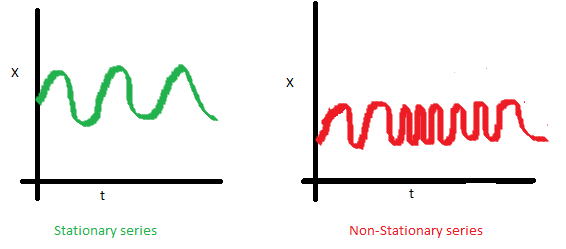一个时序数据的自协方差，就是它在不同两个时刻i,j的值的协方差。可以看到左图的自协方差于时间无关；而右图，随着时间的不同，数据的波动频率明显不同，导致它i，j取值不同，就会得到不同的协方差，因此是非稳定的。虽然右图在均值和方差上都是与时间无关的，但仍是非稳定数据。

#### 2. python判断时序数据稳定性

1.Rolling statistic-- 即每个时间段内的平均的数据均值和标准差情况。
2. Dickey-Fuller Test -- 这个比较复杂，大致意思就是在一定置信水平下，对于时序数据假设 Null hypothesis: 非稳定。
if 通过检验值(statistic)< 临界值(critical value)，则拒绝null hypothesis，即数据是稳定的；反之则是非稳定的。

from statsmodels.tsa.stattools import adfuller
def test_stationarity(timeseries):

#这里以一年为一个窗口，每一个时间t的值由它前面12个月（包括自己）的均值代替，标准差同理。
rolmean = pd.rolling_mean(timeseries,window=12)
rolstd = pd.rolling_std(timeseries, window=12)

#plot rolling statistics:
fig = plt.figure()
orig = plt.plot(timeseries, color = 'blue',label='Original')
mean = plt.plot(rolmean , color = 'red',label = 'rolling mean')
std = plt.plot(rolstd, color = 'black', label= 'Rolling standard deviation')

plt.legend(loc = 'best')
plt.title('Rolling Mean & Standard Deviation')
plt.show(block=False)

#Dickey-Fuller test:

print 'Results of Dickey-Fuller Test:'
dftest = adfuller(timeseries,autolag = 'AIC')
#dftest的输出前一项依次为检测值，p值，滞后数，使用的观测数，各个置信度下的临界值
dfoutput = pd.Series(dftest[0:4],index = ['Test Statistic','p-value','#Lags Used','Number of Observations Used'])
for key,value in dftest.items():
dfoutput['Critical value (%s)' %key] = value

print dfoutput

ts = data['#Passengers']
test_stationarity(ts)### 3. 让时序数据变成稳定的方法

1. 趋势（trend）-数据随着时间变化。比如说升高或者降低。
2. 季节性(seasonality)-数据在特定的时间段内变动。比如说节假日，或者活动导致数据的异常。

ts_log = np.log(ts)

1. 检测和去除趋势
通常有三种方法：
• 聚合 : 将时间轴缩短，以一段时间内星期/月/年的均值作为数据值。使不同时间段内的值差距缩小。
• 平滑： 以一个滑动窗口内的均值代替原来的值，为了使值之间的差距缩小
• 多项式过滤：用一个回归模型来拟合现有数据，使得数据更平滑。

Moving Average--移动平均

moving_avg = pd.rolling_mean(ts_log,12)
plt.plot(ts_log ,color = 'blue')
plt.plot(moving_avg, color='red')ts_log_moving_avg_diff = ts_log-moving_avg
ts_log_moving_avg_diff.dropna(inplace = True)
test_stationarity(ts_log_moving_avg_diff)# halflife的值决定了衰减因子alpha：  alpha = 1 - exp(log(0.5) / halflife)
expweighted_avg = pd.ewma(ts_log,halflife=12)
ts_log_ewma_diff = ts_log - expweighted_avg
test_stationarity(ts_log_ewma_diff)1. 检测和去除季节性
有两种方法：
• 1 差分化： 以特定滞后数目的时刻的值的作差
• 2 分解： 对趋势和季节性分别建模在移除它们

Differencing--差分

ts_log_diff = ts_log - ts_log.shift()
ts_log_diff.dropna(inplace=True)
test_stationarity(ts_log_diff)3.Decomposing-分解

#分解(decomposing) 可以用来把时序数据中的趋势和周期性数据都分离出来:
from statsmodels.tsa.seasonal import seasonal_decompose
def decompose(timeseries):

# 返回包含三个部分 trend（趋势部分） ， seasonal（季节性部分） 和residual (残留部分)
decomposition = seasonal_decompose(timeseries)

trend = decomposition.trend
seasonal = decomposition.seasonal
residual = decomposition.resid

plt.subplot(411)
plt.plot(ts_log, label='Original')
plt.legend(loc='best')
plt.subplot(412)
plt.plot(trend, label='Trend')
plt.legend(loc='best')
plt.subplot(413)
plt.plot(seasonal,label='Seasonality')
plt.legend(loc='best')
plt.subplot(414)
plt.plot(residual, label='Residuals')
plt.legend(loc='best')
plt.tight_layout()

return trend , seasonal, residual#消除了trend 和seasonal之后，只对residual部分作为想要的时序数据进行处理
trend , seasonal, residual = decompose(ts_log)
residual.dropna(inplace=True)
test_stationarity(residual)### 4. 对时序数据进行预测

step1： 通过ACF,PACF进行ARIMA（p，d，q）的p，q参数估计

#ACF and PACF plots:
from statsmodels.tsa.stattools import acf, pacf
lag_acf = acf(ts_log_diff, nlags=20)
lag_pacf = pacf(ts_log_diff, nlags=20, method='ols')
#Plot ACF:
plt.subplot(121)
plt.plot(lag_acf)
plt.axhline(y=0,linestyle='--',color='gray')
plt.axhline(y=-1.96/np.sqrt(len(ts_log_diff)),linestyle='--',color='gray')
plt.axhline(y=1.96/np.sqrt(len(ts_log_diff)),linestyle='--',color='gray')
plt.title('Autocorrelation Function')

#Plot PACF:
plt.subplot(122)
plt.plot(lag_pacf)
plt.axhline(y=0,linestyle='--',color='gray')
plt.axhline(y=-1.96/np.sqrt(len(ts_log_diff)),linestyle='--',color='gray')
plt.axhline(y=1.96/np.sqrt(len(ts_log_diff)),linestyle='--',color='gray')
plt.title('Partial Autocorrelation Function')
plt.tight_layout()step2： 得到参数估计值p，d，q之后，生成模型ARIMA（p，d，q）

from statsmodels.tsa.arima_model import ARIMA
model = ARIMA(ts_log, order=(2, 1, 0))
results_AR = model.fit(disp=-1)
plt.plot(ts_log_diff)
plt.plot(results_AR.fittedvalues, color='red')model = ARIMA(ts_log, order=(0, 1, 2))
results_MA = model.fit(disp=-1)
plt.plot(ts_log_diff)
plt.plot(results_MA.fittedvalues, color='red')model = ARIMA(ts_log, order=(2, 1, 2))
results_ARIMA = model.fit(disp=-1)
plt.plot(ts_log_diff)
plt.plot(results_ARIMA.fittedvalues, color='red')step3: 将模型代入原数据进行预测


#ARIMA拟合的其实是一阶差分ts_log_diff，predictions_ARIMA_diff[i]是第i个月与i-1个月的ts_log的差值。
#由于差分化有一阶滞后，所以第一个月的数据是空的，
predictions_ARIMA_diff = pd.Series(results_ARIMA.fittedvalues, copy=True)
#累加现有的diff，得到每个值与第一个月的差分（同log底的情况下）。
#即predictions_ARIMA_diff_cumsum[i] 是第i个月与第1个月的ts_log的差值。
predictions_ARIMA_diff_cumsum = predictions_ARIMA_diff.cumsum()
#先ts_log_diff => ts_log=>ts_log => ts
#先以ts_log的第一个值作为基数，复制给所有值，然后每个时刻的值累加与第一个月对应的差值(这样就解决了，第一个月diff数据为空的问题了)
#然后得到了predictions_ARIMA_log => predictions_ARIMA
predictions_ARIMA_log = pd.Series(ts_log.ix, index=ts_log.index)
predictions_ARIMA = np.exp(predictions_ARIMA_log)
plt.figure()
plt.plot(ts)
plt.plot(predictions_ARIMA)
plt.title('RMSE: %.4f'% np.sqrt(sum((predictions_ARIMA-ts)**2)/len(ts)))### 5.总结

(1). 获取被观测系统时间序列数据；
(2). 对数据绘图，观测是否为平稳时间序列；对于非平稳时间序列要先进行d阶差分运算，化为平稳时间序列；
(3). 经过第二步处理，已经得到平稳时间序列。要对平稳时间序列分别求得其自相关系数ACF 和偏自相关系数PACF，通过对自相关图和偏自相关图的分析，得到最佳的阶层 p 和阶数 q
(4). 由以上得到的d、q、p，得到ARIMA模型。然后开始对得到的模型进行模型检验。

1.判断一个时序数据是否是稳定。对应步骤(1)
2. 怎样让时序数据稳定化。对应步骤(2)
3. 使用ARIMA模型进行时序数据预测。对应步骤(3,4)

https://www.analyticsvidhya.com/blog/

posted @ 2017-05-09 21:46  geek精神  阅读(72719)  评论(37编辑  收藏  举报